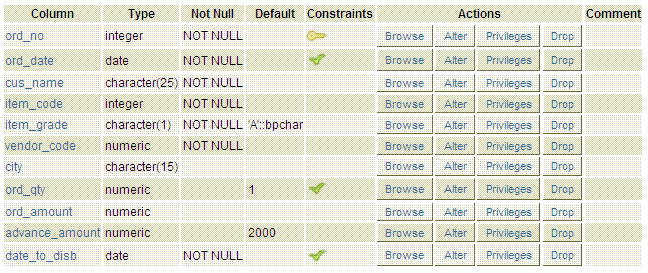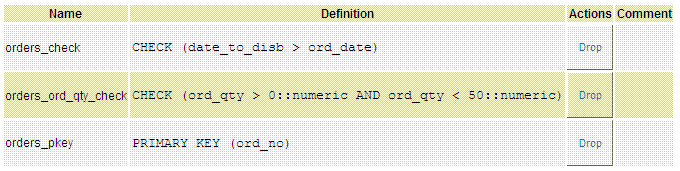# PostgreSQL ALTER TABLE

## ALTER TABLE

The PostgreSQL ALTER TABLE statement is used to change the definition or structure of an existing table. The action to be done by this statement are as follows -

• Column(s) can be dropped. If indexes and any table constraints associated the involving column will automatically be dropped. If table referenced by other table CASCADE can be used.
• Data type of column(s) can be changed and the associating indexes and constraints will automatically be converted to new column type.
• The default value for a column can be removed.

Usage

Code:

``````ALTER TABLE table_name
[action ...]
```
```

Where action is:

DROP [ COLUMN ]

ALTER [ COLUMN ] [ SET | DROP ] DEFAULT

ALTER [ COLUMN ] [ SET | DROP ] NOT NULL

PostgreSQL ALTER TABLE example

Let us consider the tables orders.

SQL

Code:

``````CREATE TABLE orders(
ord_no integer PRIMARY KEY,
ord_date date NOT NULL,
cus_name character(25) NOT NULL,
item_code integer NOT NULL,
vendor_code numeric NOT NULL,
city character(15),
ord_qty numeric DEFAULT 1 CHECK(ord_qty>0 AND ord_qty<50),
ord_amount numeric,
date_to_disb date NOT NULL CHECK(date_to_disb>ord_date)
);
```
```

The table structureThe Data DictionaryIf we want to add a column named vendor_name type varchar in orders table the following SQL can be used -

``````ALTER TABLE orders ADD COLUMN
vendor_name varchar(25);
```
```

Drop a column

If we want to drop the column vendor_name from orders table the following SQL can be used -

``````ALTER TABLE orders DROP COLUMN
vendor_name;
```
```

Change column type

If we want to change the type of column cus_name and city from character to varchar in orders table the following SQL can be used -

``````ALTER TABLE orders
ALTER COLUMN cus_name TYPE varchar(25),
ALTER COLUMN city TYPE varchar(25);
```
```

Rename a column

If we want to change the name of column city to vendor_city in orders table the following SQL can be used -

``````
ALTER TABLE orders
RENAME COLUMN city TO vendor_city;
```
```

Rename a table

If we want to change the name of an existing table the following SQL can be used -

``````ALTER TABLE orders RENAME TO neworders;
```
```

If we want to add the NOT NULL constraint to city column in orders table the following SQL can be used -

``````ALTER TABLE orders ALTER COLUMN city SET NOT NULL;
```
```

Remove NOT NULL constraint

If we want to remove the NOT NULL constraint from the city column in orders table the following SQL can be used -

``````ALTER TABLE orders ALTER COLUMN city DROP NOT NULL;
```
```

If we want to add a check constraint in orders table and all it children the following SQL can be used -

``````ALTER TABLE orders ADD CONSTRAINT chkamount CHECK (ord_amount>=10000);
```
```

Remove CHECK constraint

If we want to remove the check constraint chkamount from orders table and all it children the following SQL can be used -

``````ALTER TABLE orders DROP CONSTRAINT chkamount;
```
```

Remove CHECK constraint only from parent table

If we want to remove the check constraint chkamount from orders table and all it children the following SQL can be used -

``````ALTER TABLE ONLY orders DROP CONSTRAINT chkamount;
```
```

If we want to add a unique constraint item_vendor_ukey in orders table the following SQL can be used -

``````ALTER TABLE orders
UNIQUE (item_code,vendor_code);
```
```

Remove UNIQUE constraint

If we want to remove unique constraint from orders table the following SQL can be used -

``````ALTER TABLE orders
DROP CONSTRAINT item_vendor_ukey;
```
```

If we want to add a primary key constraint in orders table the following SQL can be used. Only one primary key is allowed for a table. So, we have to assume that we have no pre-defined primary key in the table which we are going to use.

``````ALTER TABLE orders ADD PRIMARY KEY (ord_no);
```
```

If we want to add a foreign key constraint in orders table the following SQL can be used. Assume that we have a table vendors and it contains a column vendor_code with same data type as in orders table.

``````ALTER TABLE orders
``` ```